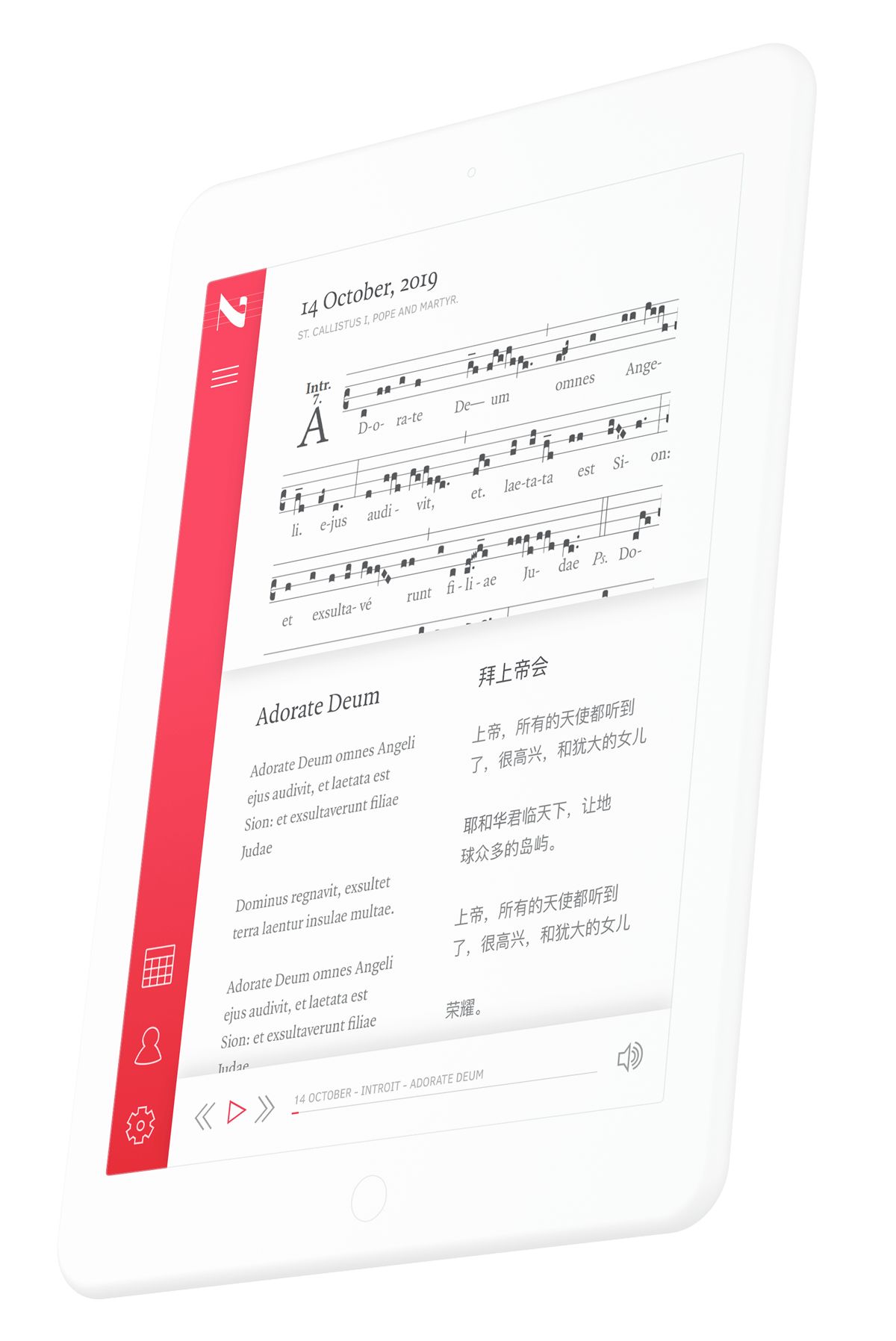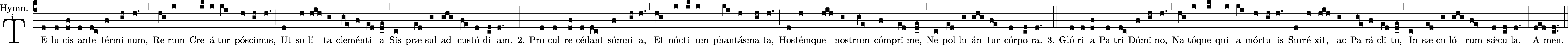# Gregorian Chant Karaoke

Scrolling with purpose.Neumz is a digital resource for the entire Gregorian chant repertory. The application features recordings by the community of Benedictine nuns of the Abbey of Notre-Dame de Fidélité of Jouques, in French Provence.

This is an exciting new project that we launched last month, and it's been full of interesting technical challenges. Here's how we tackled one of the trickier ones: a scrolling karaoke-style display that syncs up an audio recording, medieval sheet music, Latin lyrics, and those same lyrics translated into several languages.

Users can listen to these Gregorian chants while following along with its score and translation as it scrolls in time with the recording.

#### Technical Challenges

To create a score and translation, admins upload a recording file and the corresponding GABC notation. GABC is a text notation that is use to describe Gregorian chant scores.

For example, the GABC notation of Te lucis 1 - Dom Pentecostes looks like:

``````%%
initial-style: 1;
name: Te lucis ante terminum;
mode: 1;
office-part: Hymnus;
annotation: Hymn.;
annotation: i.;
%%
(c4)TE(d) lu(d)cis(df) an(d)te(dc) tér(f)mi(gh)num,(h.) (,)
Re(hg)rum(j) Cre(jk)á(j)tor(ji) pó(h)sci(gh)mus,(h.) (;)
Ut(d) so(h)lí(hih)ta(g) cle(ge)mén(f)ti(ed)a(d_e) (,)

2. Pro(d)cul(d) re(df)cé(d)dant(dc) sóm(f)ni(gh)a,(h.) (,)
Et(hg) nóc(j)ti(jk)um(j) phan(ji)tás(h)ma(gh)ta,(h.) (;)
Hos(d)tém(h)que(hih) nos(g)trum(ge) cóm(f)pri(ed)me,(d_e) (,)
Ne(c) pol(ed)lu(g)án(ghg)tur(fe) cór(d)po(cd)ra.(d.) (::)

3. Gló(d)ri(d)a(df) Pa(d)tri(dc) Dó(f)mi(gh)no,(h.) (,)
Na(hg)tó(j)que(jk) qui(j) a(ji) mór(h)tu(gh)is(h.) (;)
Sur(d)ré(h)xit,(hih) ac(g) Pa(ge)rá(f)cli(ed)to,(d_e) (,)
In(c) sæ(ed)cu(g)ló(ghg)rum(fe) sǽ(d)cu(cd)la.(d.) (::)
A(ded)men.(cd..) (::)``````

From this GABC notation, we generate a vertical and horizontal score. The horizontal score is primarily used for mobile devices, while the vertical score is best for larger screens.When a user is listening to a recording on Neumz, the score scrolls in time with the recording. A generated score in Neumz is a PNG image. This presented an interesting problem. We had to determine the location on the PNG image that corresponded with the current place in the recording. Our goal was to ensure that the correct piece of the score was always present on the screen, and that we didn't scroll too fast or too slow through certain parts of the chant.

#### Vertical Scores

The process for calculating scrolling speed for vertical scores is more complex than horizontal. The general process is as follows:

When we generate the PNG from the GABC notation, we set a restriction on the width to be 1181px. With this information, we can determine the average height of a line in the score by counting the number of lines in a generated score and dividing the width by the number of lines. This was calculated to be 227.5px.

We store the total height of the score when it is created. This information comes from the metadata of `ActiveStorage::Analyzer::ImageAnalyzer` . Using this height, we can then estimate the number of lines in the score. For example, the vertical Te lucis 1 - Dom Pentecostes component score is 1574px tall, which gives us an estimated 7 lines.

Next, we need to estimate the number of words per line of the score.

The translations for chants are broken up into blocks which are associated with particular start and end times in the recording. The timings are adjusted by the admin so that the text translation can properly match the tempo of the chant.

Using the number of lines in the score, as well as the number of words per translation block, and the duration of the block, we can calculate the average number of words per line. In the example of the Te lucis 1 - Dom Pentecostes component, there are a total of 43 words in the translation. Divided by the estimated 7 lines, that's about 6 words per line.

Now that we know the average number of words per line, we can calculate the estimated number of lines a translation block uses by dividing the number of words in a translation block by the average words per line. Using the line "Et nóctium phantásmata," from the example Te lucis 1 - Dom Pentecostes component, we would have 3/6, or 0.5.

To find the position of the score on the page, we first look at the current translation block that is playing and sum the average number of lines a translation block uses for all previous translation blocks. For the example above, the sum of the number of lines for all previous translations is 3.12. So we are estimating that "Et nóctium phantásmata," begins partway through the 3rd line of the score.

Now that we know which line of the score we are playing, we can figure out how far through the score we are. Since we know that a line is 227.5px tall, we can multiply 227.5 by the line number 3 to get 628.5px, which is the position of the image that we want to scroll to. If we divide 628.5px by the total score height of 1574px, we get 0.208, which means that we're 40% through the score, and so that's where we scroll to.

Once we've calculated the base scroll position of 40%, we can figure out how far it is to the next translation by repeating the calculations above to figure out what the percentage of the next translation block. Let's say that in this example, the next percentage is 50%. We're able to calculate the scroll speed by taking the difference of the two percentages, 10%, and dividing that by the duration of the translation block, 6.9 seconds, and we know how fast to scroll per second so that we are in the correct position for the next translation block.

The previous method for calculating the position on the page would divide the current position of the recording by the total duration of the recording. This would often cause the text in the score to be off the page because the duration of a block and the length of text varied significantly.

#### Horizontal Scores

For horizontal scores, the process is very similar to vertical scores, except that we do not need to take into account the height of the score. Instead, we use the width of the score and divide that by the number of words in the translation to calculate the average number of pixels per word. Theoretically, this would be about the same for all scores, but we do it for each score individually.

We can calculate the width of an individual translation block by multiplying the average number of pixels per word by the number of words in the block.

From there, the percentage through the score is just the width of all previous blocks divided by the width of the score.

To calculate how fast to move the score within a block, we use the duration, start, and end time of the block as well as the current time of the recording. These values allow us to calculate what percentage we are through the chant. This is done in the same way that the vertical score offset is calculated.

Neumz is a unique project in all aspects. This scrolling feature is one of many fun technical challenges that we've worked through. Generating the score from GABC and then transcribing the recording were some of the other unique problems that we hadn't solved before this project. It has been great seeing the application in action as the first digital resource for the entire Gregorian chant repertory.

If you want to check out the scrolling in action, or maybe you just want some background music at the office, Neumz is live at app.neumz.com!